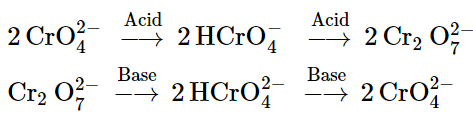# Describe the preparation of potassium dichromate from iron chromite ore.Question:

Describe the preparation of potassium dichromate from iron chromite ore. What is the effect of increasing pH on a solution of potassium dichromate?

Solution:

Potassium dichromate is prepared from chromite ore $\left(\mathrm{FeCr}_{2} \mathrm{O}_{4}\right)$ in the following steps.

Step (1): Preparation of sodium chromate

$4 \mathrm{FeCr}_{2} \mathrm{O}_{4}+16 \mathrm{NaOH}+7 \mathrm{O}_{2} \longrightarrow 8 \mathrm{Na}_{2} \mathrm{CrO}_{4}+2 \mathrm{Fe}_{2} \mathrm{O}_{3}+8 \mathrm{H}_{2} \mathrm{O}$

Step (2): Conversion of sodium chromate into sodium dichromate

$2 \mathrm{Na}_{2} \mathrm{CrO}_{4}+$ conc. $\mathrm{H}_{2} \mathrm{SO}_{4} \longrightarrow \mathrm{Na}_{2} \mathrm{Cr}_{2} \mathrm{O}_{7}+\mathrm{Na}_{2} \mathrm{SO}_{4}+\mathrm{H}_{2} \mathrm{O}$

Step(3): Conversion of sodium dichromate to potassium dichromate

$\mathrm{Na}_{2} \mathrm{Cr}_{2} \mathrm{O}_{7}+2 \mathrm{KCl} \longrightarrow \mathrm{K}_{2} \mathrm{Cr}_{2} \mathrm{O}_{7}+2 \mathrm{NaCl}$

Potassium dichromate being less soluble than sodium chloride is obtained in the form of orange coloured crystals and can be removed by filtration.

The dichromate ion $\left(\mathrm{Cr}_{2} \mathrm{O}_{7}^{2-}\right)$ exists in equilibrium with chromate $\left(\mathrm{CrO}_{4}^{2-}\right)$ ion at $\mathrm{pH} 4$. However, by changing the $\mathrm{pH}$, they can be interconverted.